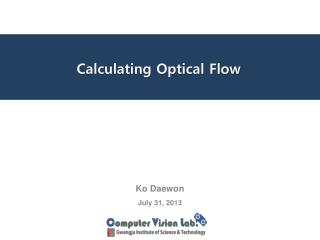DownloadDownload PresentationCalculating Optical Flow

# Calculating Optical Flow

Télécharger la présentation## Calculating Optical Flow

- - - - - - - - - - - - - - - - - - - - - - - - - - - E N D - - - - - - - - - - - - - - - - - - - - - - - - - - -
##### Presentation Transcript

1. Calculating Optical Flow KoDaewon July 31, 2013

2. Contents • Motion fields and Optical flow • What is Optical flow? • Brightness Constancy • Optical Flow Constraint Equation • Aperture problem • A Global Method: Horn-Schunck`s Method

3. 1. Motion Fields and Optical Flow Motion is one of the most important research topics in computer vision. There are several important issue to investigate: - 2D and 3D motion representations - calculating 2D motion - inferring 3D motion - structure and motion

4. 1. Motion Fields and Optical Flow A critical but difficult problem for motion analysis - Constructing correspondences ! Correspondences could be in totally different forms - point correspondences - line correspondences - curve correspondences - region correspondences

5. 1. Motion Fields and Optical Flow To solve this problem there are two major methodologies: 1) “dense” approach - Trying to build correspondences pixel by pixel. 2) “sparse” or “feature-based” approach - Trying to associate different image features.

6. Velocity vectors 2. What is Optical Flow? Unfortunately, we can not observe motion field directly, since we have no idea of how the image projection of a 3D point moves. What we can observe are only image points. What we can say is that an image point moves from here to there, which indicates optical flow. Optical Flow

7. 2. What is Optical Flow? Motion field Optical Flow

8. 2. What is Optical Flow? I(x, y, t+1) I(x, y, t) • Given two subsequent frames, calculate the velocity vectors between them. • Key assumptions - Brightness constancy : projection of the same point looks the same in every frame

9. 3. Brightness Constancy • Brightness Constancy

10. 4. Optical Flow Constraint Equation : velocity of an image pixel By Brightness constancy during dt, If the brightness changes smoothly with x, y and t, we expand the left-hand-side by Taylor series: +++O( = So, we have xx (xxx

11. 4. Optical Flow Constraint Equation We call this equation optical flow constraint equation

12. 5. Aperture problem Many points around p have the same intensity. It is impossible to determine which point on match point on . To determine the optical flow uniquely, We need some other constraints.

13. 6. A Global Method: Horn-Schunck`s Method =the variation of the optical flow field can not too big. Smoothness term = Error of optical flow = The assumption in this method: optical flow varies smoothly

14. 6. A Global Method: Horn-Schunck`s Method Goal: To find functions u and v that minimize e e(u,v) = = = + = We use the Euler-Lagrange equation (= condtion that f has a extremum)

15. 6. A Global Method: Horn-Schunck`s Method Where: + + + + = = , = = , =

16. 6. A Global Method: Horn-Schunck`s Method Since = we can rewrite the Euler-Lagrange equations as: + + = + + = Using - and - we get (+ ) + = ( - ) + (+ ) = ( - ) we can solve for and as: (+ +) = ()-- (+ +) = --

17. 6. A Global Method: Horn-Schunck`s Method Which can be written as: (+ +) ( -) = -+ (+ +) ( - ) = -+ These equations suggest an iterative scheme: = - = - k: iteration number , and : neighborhood averages of and